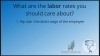# Invoicing - Part 4: How to Calculate Profit Margins## Description

So now for the fun part-- making the money! 💰

Tonya from The Profit Constructors and Duane from ClockShark take you through the difference between markups and margins and how to determin markups on billable rates and create wide and profitable margins!

Next up, check out part 5 of the invoicing series to learn about how to use crew base rates to make quick estimates on the job.

## Transcript

We are now better off after learning all the different rates.

So we've got pay rate, cost rate, and billable rate, which is the one we really want to focus on.

But where does that translate? Like, where does that mean at the end of the day? Where do I get to see the money that comes from having those rates in place and knowing what's going on?

Knowing the difference between markup and margin. I think that's where this starts to really make a lot of sense is if we hone in on what is the difference between markup and margin.

A lot of times, just like with pay rate, cost rate and billing rate that we had previously talked about, the markup and margin get used as the same word. They're not the same thing at all. So I want to mark something up. So if I know that my cost rate is \$30 for an easy number to use. So if I know that my cost rate for my guys out on the job is \$30 an hour, I want to mark that up. Let's say 50%. If I'm going to do a markup of 50%, that means my billing rate will be \$45 an hour, for some super simple math, because I'm going to take that \$30 and add 50%. Now, in order to understand my margin, that margin is not going to be a 50% margin once I've added that mark up. The margin is my gross profit divided by my revenue.

What's the difference in profit and revenue for this?

Revenue is that top line that I'm bringing in. So in this one, our it's going to be \$45 and that's billing rate, that's twice the markup, everything. So if we're billing for \$30 now, billing for \$30 an hour, 50% markup on labor. now we're at 45. So revenue is \$45 an hour. But my profit is only what's down underneath the costs. So when I subtract that \$30 of cost that I had, then what's left is \$15 of profit. That's gross profit.

Just to be clear, that's probably not what we have at the end of the day. So we're talking about we have overhead and other things you have to do. Now, if I divide that gross profit by the revenue, I'm left with 33% margin. So I marked it up 50%.

Oh, OK. I get it now because 33%, the \$15 is a third, a 45, which \$45 in total revenue. Got it.